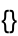#Function Repository Resource:

# IntersectionBasis

Find a basis for the intersection of subspaces of ℝ^n

Contributed by: Dennis M Schneider
 ResourceFunction["IntersectionBasis"][list1,list2,…] finds a basis for the intersection of the subspaces of ℝn spanned by list1,list2, ….

## Details and Options

The method is based on expressing each subspace as the null space of a matrix and then finding the null space of the matrix obtained by stacking these matrices vertically.
The empty list spans the trivial subspace.

## Examples

### Basic Examples

The intersection of two three-dimensional subspaces of 4:

 In:=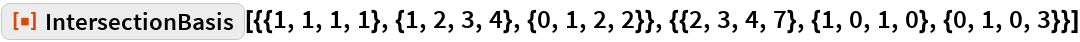Out=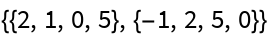Two subspaces are called disjoint if their intersection is the trivial space, and a basis for the trivial subspace is the empty list. These two subspaces are disjoint:

 In:=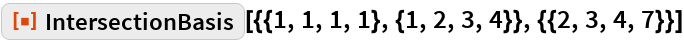Out=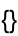All vectors in the lists must have the same size:

 In:=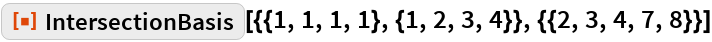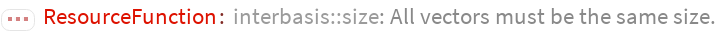Out=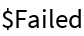Again, the vectors in the list must have the same size; the sizes of the vectors in the second list are 4 and 0:

 In:=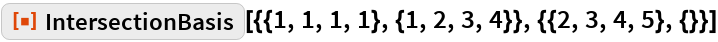Out=Compare with the following. The first list has two elements, the second has one, and the third also has one:

 In:=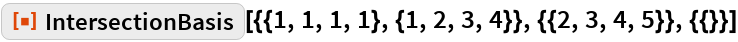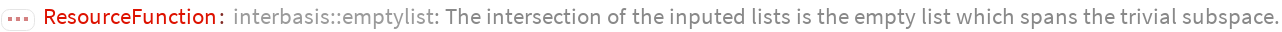Out=